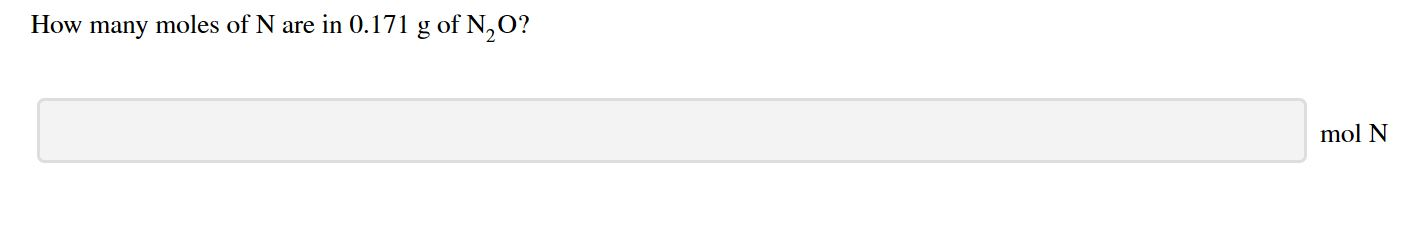# moles of N are in 0.171 g of N0?Howmanymol N

Question
25 viewshelp_outlineImage Transcriptionclosemoles of N are in 0.171 g of N0? How many mol N fullscreen
check_circle

Step 1

According to the questions,

Mass of N2O = 0.171 g

Molar mass of N2O = 44.013 g/mol

As per the mole concept:

Step 2

Thus, the number of moles of N2O in 0.171 g will be calculated as follows:

Step 3

As per the molecular formula of dinitrogen monoxide that is N2O, 1 mol N2O conatins 2 mol N, the...

### Want to see the full answer?

See Solution

#### Want to see this answer and more?

Solutions are written by subject experts who are available 24/7. Questions are typically answered within 1 hour.*

See Solution
*Response times may vary by subject and question.
Tagged in

### Chemistry